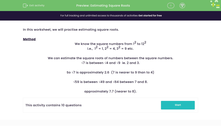# Estimate Square Roots

In this worksheet, students will practise estimating square roots.Key stage:  KS 3

Curriculum topic:   Number

Curriculum subtopic:   Understand Integer Powers/Real Roots

Popular topics:   Numbers worksheets, Estimation worksheets, Square Numbers worksheets

Difficulty level:#### Worksheet Overview

In this worksheet, we will practise estimating square roots.

This table shows the first few whole numbers and their squares.

 1 2 3 4 5 6 7 8 9 10 1 4 9 16 25 36 49 64 81 100

This means that the √81 = 9 and √100 = 10.

It also means that the square root of any number between 81 and 100 lies between 9 and 10.

We can estimate the square roots of numbers between the square numbers.

√7 is between √4 and √9  ie. 2 and 3.

So √7 is approximately 2.6  (7 is nearer to 9 than to 4)

√59 is between √49 and √64 between 7 and 8.

It is nearer to 8, so we could say it is approximately 7.7

Does that all make sense?Let's have a go at some questions.

### What is EdPlace?

We're your National Curriculum aligned online education content provider helping each child succeed in English, maths and science from year 1 to GCSE. With an EdPlace account you’ll be able to track and measure progress, helping each child achieve their best. We build confidence and attainment by personalising each child’s learning at a level that suits them.

Get started